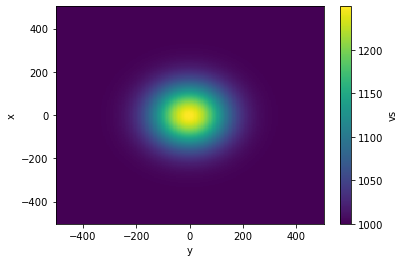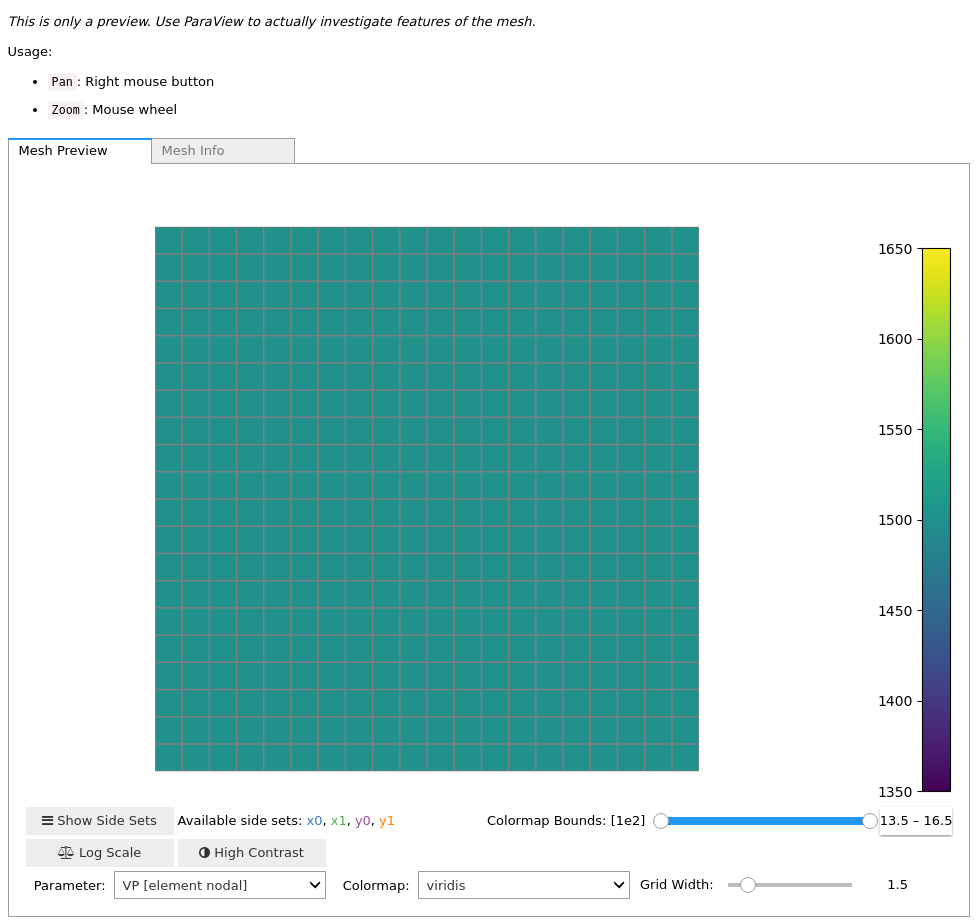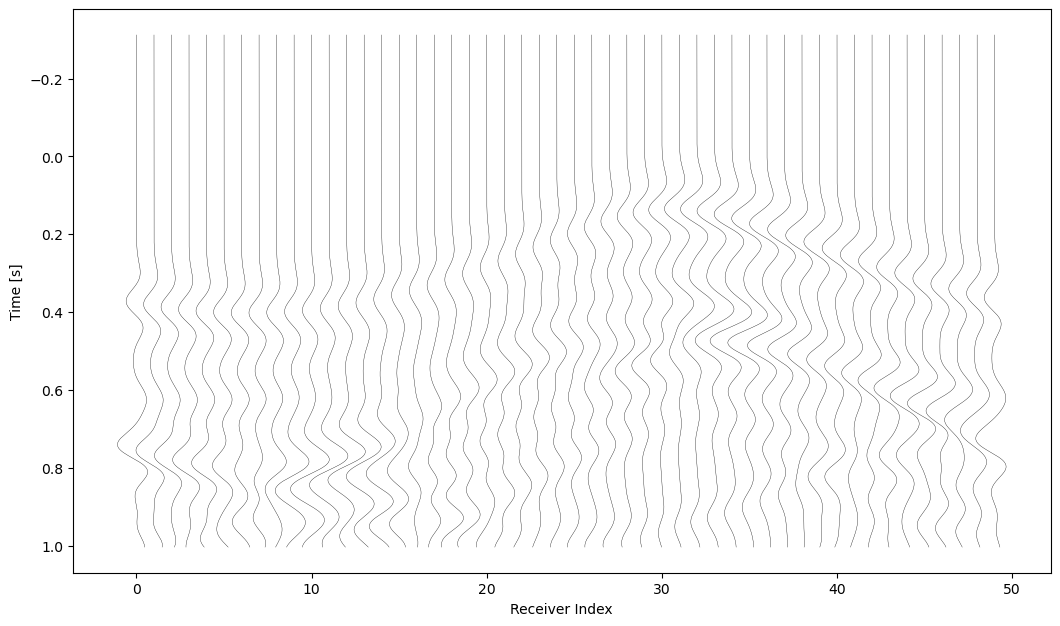Version:

This documentation is not for the latest stable Salvus version.This tutorial is presented as Python code running inside a Jupyter Notebook, the recommended way to use Salvus. To run it yourself you can copy/type each individual cell or directly download the full notebook, including all required files.# Manual Gradient Computation

This notebook teaches how to compute gradients with respect to a mesh's material parameters using only SalvusFlow and not SalvusProject. SalvusProject internally handles many of the complexities but the approach presented here grants a bit more flexibility.

Copy
# Variable used in the notebook to determine which site
# is used to run the simulations.
import os
SALVUS_FLOW_SITE_NAME = os.environ.get("SITE_NAME", "local")
%matplotlib inline

import matplotlib.pyplot as plt
import numpy as np
import pathlib
import xarray as xr

import salvus.namespace as sn

## Step 1: Generate target and initial model

This example is purely synthetic so we'll generate a target model with a Gaussian blob perturbation in $v_s$ in the center of the domain. This will be used to generate the "observed data" in this case. The initial model (the synthetics for cause of this tutorial) is a homogeneous model without the blob.

# Use xarray to define the blob in vs.
def get_target_model():
x = np.linspace(-500, 500, 200)
y = np.linspace(-500, 500, 200)
xx, yy = np.meshgrid(x, y, indexing="ij")

# Simple gaussian blob around the center of the domain.
d = (xx ** 2 + yy ** 2) ** 0.5
vs = np.exp(-0.5 * (d / 100.0) ** 2) * 250.0 + 1000.0

ds = xr.Dataset(
data_vars={"vs": (["x", "y"], vs)}, coords={"x": x, "y": y}
)

return ds

target_model = get_target_model()
target_model.vs.plot()
<matplotlib.collections.QuadMesh at 0x7fa4630d8e50>Create a mesh - this is directly used as the initial model.

# Build mesh for the initial model.
initial_model_mesh = sn.simple_mesh.CartesianHomogeneousIsotropicElastic2D(
vp=1500.0,
vs=1000.0,
rho=2000.0,
x_max=float(target_model.x.data.ptp()),
y_max=float(target_model.y.data.ptp()),
max_frequency=10.0,
tensor_order=1,
).create_mesh()

# Shift.
initial_model_mesh.points[:, 0] += target_model.x.data.min()
initial_model_mesh.points[:, 1] += target_model.y.data.min()

Now interpolate the blob onto it thus creating the target mesh. Have a look at the perturbation in $v_s$.

target_model_mesh = initial_model_mesh.copy()

target_model_mesh = sn.toolbox.interpolate_cartesian(
data=target_model, mesh=target_model_mesh, extrapolate=True
)
target_model_mesh<salvus.mesh.unstructured_mesh.UnstructuredMesh at 0x7fa462f94b90>

This is also the starting point for setting up a project for the inversion. We can directly create the project from the model defined above. We will call this model the true_model.

## Step 2: Setup the simulation with a single source and a ring of receivers

# Explosive source in one corner of the mesh.
src = sn.simple_config.source.cartesian.MomentTensorPoint2D(
mxx=10000.0,
myy=10000.0,
mxy=0.0,
x=300.0,
y=300.0,
source_time_function=sn.simple_config.stf.Ricker(center_frequency=5.0),
)

# A centered ring of receivers.
recs = sn.simple_config.receiver.cartesian.collections.RingPoint2D(
x=0, y=0, radius=200.0, count=50, fields=["displacement"]
)

# Create two simulation objects.
# (1) The target will serve as observed data.
w_target = sn.simple_config.simulation.Waveform(
mesh=target_model_mesh, sources=src, receivers=recs
)
w_target.physics.wave_equation.end_time_in_seconds = 1.0
w_target

# (2) The initial model will be used to compute synthetics.
w_initial = sn.simple_config.simulation.Waveform(
mesh=initial_model_mesh, sources=src, receivers=recs
)
w_initial.physics.wave_equation.end_time_in_seconds = 1.0

# Must store the checkpoints for the subsequent adjoint run.
w_initial.output.volume_data.format = "hdf5"
w_initial.output.volume_data.filename = "output.h5"
w_initial.output.volume_data.fields = ["adjoint-checkpoint"]
w_initial.output.volume_data.sampling_interval_in_time_steps = (
"auto-for-checkpointing"
)

## Step 3: Run Simulations

For the target/observed model and the initial/current model.

# Create the "observed data".
j_target = sn.api.run(
input_file=w_target,
site_name=SALVUS_FLOW_SITE_NAME,
output_folder="output_target",
overwrite=True,
)

# Forward run for the synthetics.
j_initial = sn.api.run(
input_file=w_initial,
site_name=SALVUS_FLOW_SITE_NAME,
output_folder="output_initial",
overwrite=True,
# Don't delete the remote files as they are needed
# for the adjoint run!
delete_remote_files=False,
)
Job job_2103021244581427_4b2387448c running on local with 4 rank(s).
Site information:
* Salvus version: 0.11.27
* Floating point size: 32
-> Current Task: Time loop complete* Downloaded 342.0 KB of results to output_target.
* Total run time: 0.91 seconds.
* Pure simulation time: 0.52 seconds.
Job job_2103021244396726_9463612a9b running on local with 4 rank(s).
Site information:
* Salvus version: 0.11.27
* Floating point size: 32
-> Current Task: Time loop complete* Downloaded 342.4 KB of results to output_initial.
* Total run time: 1.06 seconds.
* Pure simulation time: 0.72 seconds.

# Load the data from both runs, and visualize.
#
# Note that this is a vectorial receiver and thus the component
# has to be specified.
target_event = j_target.get_as_event()
initial_event = j_initial.get_as_event()

target_event.plot(receiver_field="displacement", component="X")
initial_event.plot(receiver_field="displacement", component="X")